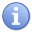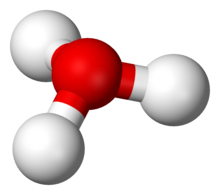# Сборник текстов на казахском, русском, английском языках для формирования навыков по видам речевой деятельности обучающихся уровней среднего образования

 бет 41/65 Дата 05.11.2016 өлшемі 17,36 Mb.

## Amphoterism and Water

Substances capable of acting as either an acid or a base are amphoteric. Water is the most important amphoteric substance. It can ionize into hydroxide (OH-, a base) or hydronium (H3O+, an acid). By doing so, water is

1. Increasing the H+ or OH- concentration (Arrhenius),

2. Donating or accepting a proton (Brønsted-Lowry), and

3. Accepting or donating an electron pair (Lewis).Important A bare proton (H+ ion) cannot exist in water. It will form a hydrogen bond to the nearest water molecule, creating thehydronium ion (H3O+). Although many equations and definitions may refer to the "concentration of H+ ions", that is a misleading abbreviation. Technically, there are no H+ ions, only hydronium (H3O+) ions. Fortunately, the number of hydronium ions formed is exactly equal to the number of hydrogen ions, so the two can be used interchangeably.H+ ions actually exist as hydronium, H3O+.

Water will dissociate very slightly (which further explains its amphoteric properties).
 {\displaystyle {\hbox{H}}_{2}{\hbox{O}}\leftrightarrow {\hbox{H}}^{+}+{\hbox{OH}}^{-}} The presence of hydrogen ions indicates an acid, whereas the presence of hydroxide ions indicates a base. Being neutral, water dissociates into both equally. {\displaystyle 2{\hbox{H}}_{2}{\hbox{O}}\leftrightarrow {\hbox{H}}_{3}{\hbox{O}}^{+}+{\hbox{OH}}^{-}} This equation is more accurate—hydrogen ions do not exist in water because they bond to form hydronium. Helpful Hint! Although the other halogens make strong acids, hydrofluoric acid (HF) is a weak acid. Despite being weak, it is incredibly corrosive—hydrofluoric acid dissolves glass and metal!

Most acids and bases are weak. You should be familiar with the most common strong acids and assume that any other acids are weak.

 Formula Strong Acid HClO4 Perchloric acid HNO3 Nitric acid H2SO4 Sulfuric acid HCl, HBr, HI Hydrohalic acids

Within a series of oxyacids, the ions with the greatest number of oxygen molecules are the strongest. For example, nitric acid (HNO3) is strong, but nitrous acid (HNO2) is weak. Perchloric acid (HClO4) is stronger than chloric acid (HClO3), which is stronger than the weak chlorous acid (HClO2). Hypochlorous acid (HClO) is the weakest of the four.

Common strong bases are the hydroxides of Group 1 and most Group 2 metals. For example, potassium hydroxide and calcium hydroxide are some of the strongest bases. You can assume that any other bases (including ammonia and ammonium hydroxide) are weak.

 Formula Strong Base LiOH Lithium hydroxide NaOH Sodium hydroxide KOH Potassium hydroxide RbOH Rubidium hydroxide CsOH Cesium hydroxide Ca(OH)2 Calcium hydroxide Sr(OH)2 Strontium hydroxide Ba(OH)2 Barium hydroxide

 Acids and bases that are strong are not necessarily concentrated, and weak acids/bases are not necessarily dilute. Concentration has nothing to do with the ability of a substance to dissociate. Furthermore, polyprotic acids are not necessarily stronger than monoprotic acids.

Достарыңызбен бөлісу:

©engime.org 2020
әкімшілігінің қараңыз

Басты бет